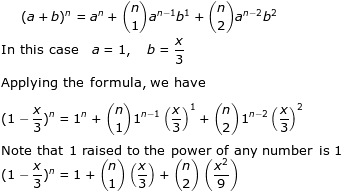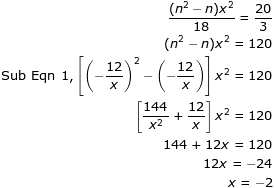### Binomial Theorem

Question

If the first 3 terms of the expansion ofare, find the value of x and n

Solution

Recall the binomial expansion formulaNext we need to simplifyGoing back to the question,We can then form 2 equations involving n and x

Equation 1Equation 21.Alternatively, from Eqn (2):

(nx)^2 - nx * x = 120.

Also, from Eqn (1),
nx = -12, (nx)^2 = 144,
144 - (-12)x = 120
12x = -24
x = -2

This method will avoid denominator..

2.One just has to be good at the basic mathematical operations, like multiplication, addition and subtraction to understand Vedic maths. Believe us, Vedic maths is this easy.
supremedissertation

3.This comment has been removed by the author.